﻿ 3.3 Spot Rates and the Yield to Maturityoffice (412) 967-9367
fax (412) 967-5958
toll-free 1 (800) 214-3480

3.3  American Call Option:  Zero-Dividend Case

 T

he difference between an American option and its European counterpart is the right to early exercise.  The American option can be exercised at any time; the European option can be exercised only at maturity.

This complicates the valuation problem, because we now have to determine whether the right to exercise early is worth something.  If it is, then the option price must reflect this additional value.  Note that an American option is never worth less than a European option.  This is because you can always choose not to exercise early.

It turns out that whether the early exercise possibility is valuable depends on whether we have a put or a call option, and whether the stock pays a dividend during the life of the option.

In this topic, you will see that the right to early exercise for an American call option, defined on a non-dividend-paying security, is zero.  To see why, let us revisit the one-period binomial model.

By applying the risk-neutral valuation principle, the present value of the call times r (i.e., Cr) in the one-period model is: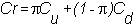where  p is the risk-neutral probability (see topic 2.7, Risk-Neutral Valuation Principle in Chapter 2), and r  is  one plus the interest rate.  In the one-period model, Cu and Cd are the terminal call option values.  In other words, Cu = max{Su-X,0}, Cd = max{Sd-X,0}.

We can demonstrate that the right to early exercise has zero value.  By substituting Cu and Cd into the risk-neutral valuation equation, we get:Adding and subtracting X to this yields: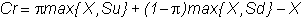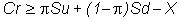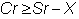Dividing both the left-hand and right-hand sides by r implies: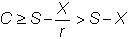because X/r < X since r > 1.

This demonstrates that the value of the call is always greater than what could be obtained by exercising the call (i.e., S - X), so the call option should never be exercised prior to maturity.

Therefore the value of the right to the early exercise of an American call option on a zero-dividend-paying stock is zero.  You will see that this does not hold for the American put option, so early exercise may indeed be called for.  This is covered in the next  topic American Put Option:  Zero-Dividend Case.